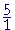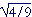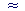Topics in

P R E C A L C U L U S

2

# RATIONAL AND IRRATIONAL NUMBERS

What is a rational number?

CALCULUS IS A THEORY OF MEASUREMENT. The necessary numbers are the rationals and irrationals. But let us start at the beginning.

The following numbers of arithmetic are the counting-numbers or, as they are called, the natural numbers:

1,  2,  3,  4,  and so on.

(At any rate, those are their Arabic numerals.)

If we include 0, we have the whole numbers:

0,  1,  2,  3,  and so on.

And if we include their algebraic negatives, we have the integers:

0,  ±1,  ±2,  ±3,  and so on.

± ("plus or minus") is called the double sign.

The following are the square numbers, or the perfect squares:

1   4   9   16   25   36   49   64, and so on.

They are the numbers 1· 1,  2· 2,  3· 3,  4· 4,  and so on.

Rational and irrational numbers

1.  What is a rational number?

A rational number is simply any number of arithmetic: Any whole number, fraction, mixed number, or decimal; together with its negative image.

2.  Which of the following numbers are rational?

 1 −1 0 23 − 23 5½ −5½ 6.085 −6.085 3.14159

To see the answer, pass your mouse over the colored area.
To cover the answer again, click "Refresh" ("Reload").

All of them. All decimals are rational. That long one is an approximation to π, which, as we shall see, is not equal to any decimal. For if it were, it would be rational.

3.   A rational number can always be written in what form?

 As a fraction ab , where a and b are integers (b0).

That is the formal definition of a rational number. That is how we can make any number of arithmetic look. An integer itself can be written as a fraction: 5 =.  And from arithmetic, we know that we can write a decimal as a fraction.

When a and b are natural numbers, then we can always name the ratio that the fraction has to 1 and which the numerator has to the denominator. Hence the name, rational number.

(is to 1 as 2 is to 3.  2 is two thirds of 3.is two thirds of 1.)

The language of arithmetic is ratio. It is the language with which we relate rational numbers to one another and to 1, which is their source. The whole numbers are the multiples of 1, the fractions are its parts: its halves, thirds, fourths, fifths, millionths. But we will see that language cannot express the relationship of an irrational number to 1

Finally, we can in principle (by Euclid VI, 9) place any rational number exactly on the number line.We can say that we truly know a rational number.

WHY DO WE EVEN CALL numbers "rational"? Because there are numbers that are not rational. For example,("Square root of 2"). It is not possible to name any number of arithmetic—any whole number, any fraction, or any decimal—whose square is 2.is close because

 75 · 75 = 4925

—which is almost 2.

To prove that there is no rational number whose square is 2, suppose there were. Then we could express it as a fractionin lowest terms. That is, suppose·== 2.

But that is impossible. Sinceis in lowest terms, then m and n have no common divisors except 1. Therefore, m·m and n·n also have no common divisors, and it will be impossible to divide n·n into m·m and get 2.

There is no rational number—no number of arithmetic—whose square is 2.  Therefore we callan irrational number.

By recalling the Pythagorean theorem, we can see that irrationalnumbers are necessary.  For if the sides of an isosceles right triangle are called 1, then we will have  12 + 12 = 2, so that the hypotenuse is.  There really is a length that logically deserves the name, "."  Inasmuch as numbers name the lengths of lines, thenis a number.

4.  Which natural numbers have rational square roots?

Only the square roots of the square numbers; that is, the square roots of the perfect squares.= 1  RationalIrrationalIrrational= 2  Rational,,,Irrational= 3  Rational

And so on.

The square roots of the square numbers are the only square roots that we can name.  That follows from the same proof thatis irrational.

Not every length, then, can be named by a rational number. Pythagoras realized that in the 6th century B.C.He realized that the relationship of the diagonal of a square to the side was not as two natural numbers—which we can always name. That relationshp, he said, was without a name. For if we ask, "What relationship has the diagonal to the side?"—we cannot say. Nowadays, of course, we call it "Square root of 2.." But the idea of an irrational number had not yet occurred. It was not until many centuries after Pythagoras that the radical sign was created.

Irrational numbers have been called surds, after the Latin surdus, deaf or mute. Why deaf or mute? Because there is nothing we can hear. An irrational number cannot say how much it is, nor how it is related to 1. An irrational number and 1 are incommensurable.

5.  Say the name of each number.

a)"Square root of 3."

b)"Square root of 5."

c)"2."  This is a rational—nameable—number.

d)"Square root of 3/5."

e)"2/3."

In the same way we saw that only the square roots of square numbers are rational, we could prove that only the nth roots of nth powers are rational. Thus, the 5th root of 32 is rational, because 32 is a 5th power, namely the 5th power of 2. But the 5th root of 33 is irrational. 33 is not a perfect 5th power.

The decimal representation of irrationals

When we express a rational number as a decimal, then either the decimal will be exact, as= .25, or it will not be, as.3333.  Nevertheless, there will be a predictable pattern of digits.  But when we express an irrational number as a decimal, then clearly it will not be exact, because it were, the number would be rational.

Moreover, there will not be a predictable pattern of digits.  For example,1.4142135623730950488016887242097

(This symbolmeans "is approximately.")

Now, with rational numbers, you sometimes see= .090909. . .

By writing both the equal sign = and three dots (ellipsis) we mean:

"It is not possible to expressexactly as a decimal. However, we can approximate it with as many decimal digits as we please according to the indicated pattern; and the more decimal digits we write, the closer we will be to."

We say that any decimal foris inexact.  But the decimal for, which is .25, is exact.

The decimal for an irrational number is always inexact.  An example is the decimal forabove.

If we write ellipsis—= 1.41421356237. . .

—we mean:

No decimal forwill be exact. Moreover, there will not be a predictable pattern of digits. We could continue its rational approximation for as many decimal digits as we please by means of the algorithm, or method, for calculating each next digit (not the subject of these Topics); and again, the more digits we calculate, the closer we will be to.

It is important to understand that no decimal that you or anyone will ever see  is equal to, or π, or any irrational number. We know an irrational number only as a rational approximation. And if we choose a decimal approximation, then the more decimal digits we calculate, the closer we will be to the value.

(For a decimal approximation of π, see Topic 9 of Trigonometry.)

To sum up, a rational number is a number we can know and name exactly, either as a whole number, a fraction, or a mixed number, but not always exactly as a decimal.  An irrational number we can never know exactly in any form.

Real numbers

5.  What is a real number?

A real number is distinguished from an imaginary or complex number. It is what we call any rational or irrational number.
They are the numbers we expect to find on the number line.
They are the numbers we need for measuring.

(An actual measurement can result only in a rational number.
An irrational number can result only from a theoretical  calculation or a definition. Examples of calculations are the Pythagorean theorem and the solution to an equation, such as x3 = 5.  The irrational number π is defined as the ratio of the circumference of a circle to the diameter.)

Problem 1.   We have categorized numbers as real, rational, irrational, and integer.  Name all the categories to which each of the following belongs.

 a) 3  Real, rational, integer. b) −3  Real, rational, integer. c)−½  Real, rational. d);Real, irrational. e) 5¾  Real, rational. f) − 11/2  Real, rational. g) 1.732  Real, rational. h) 6.920920920. . .  Real, rational. i) 6.92057263. . .   Real. And let us assume that it is irrational, that is, no matter how many digits we calculate, they do not repeat. We must assume, therefore, that there is a procedure—a rule—for computing each next digit. For if there were not, then we would not know that symbol's position with respect to order. We would not be able to decide whether it is less than or greater than 6.920572635. Which is to say, it would not be a number. (See Are the real numbers really numbers?) j) 6.920572635   Real, rational. Every exact decimal is rational.

7.  What is a real variable?

A variable is a symbol that takes on values. A value is a number.
A real variable takes on values that are real numbers.

Calculus is the study of functions of a real variable.

Problem 2.   Let x be a real variable, and let 3 < x < 4.  Name five values that x might have.*

See The Evolution of the Real Numbers starting with the natural numbers.Next Topic:  Functions

Please make a donation to keep TheMathPage online.
Even \$1 will help.